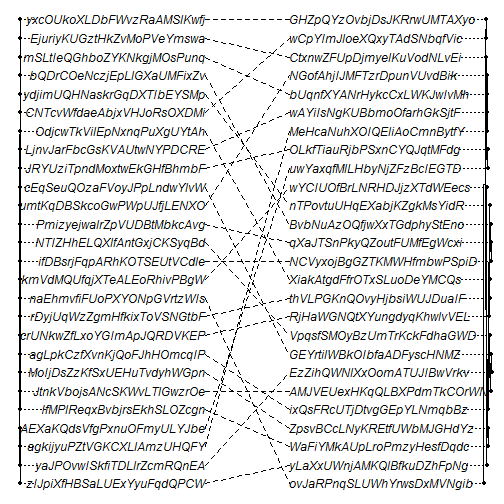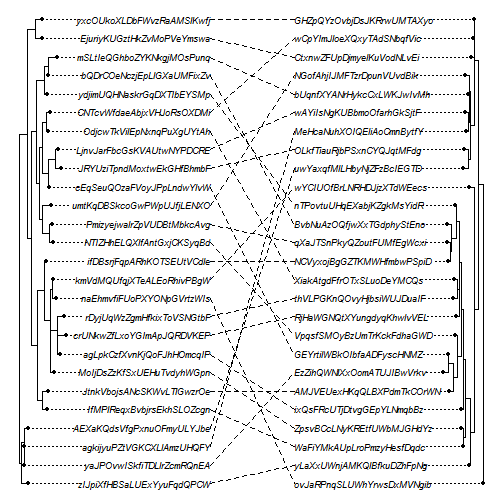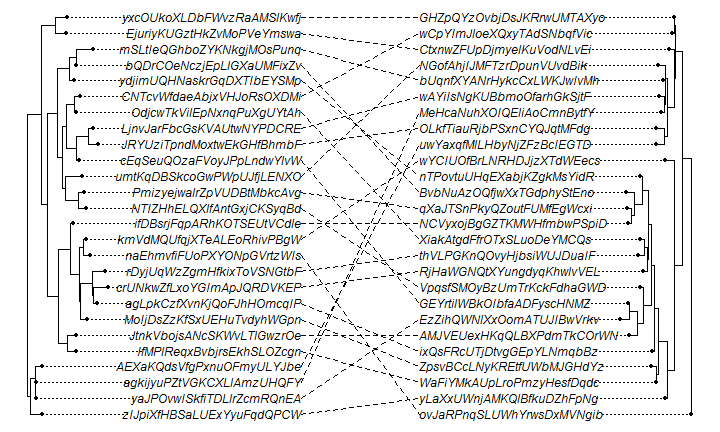## Wednesday, February 3, 2016

### `plot.cophylo` for very long tip labels

On the R-sig-phylo list a subscriber reported some weird behavior from the phytools plotting method for objects of class `"cophylo"`. I don't have the original dataset, but it looked something like:

``````obj<-cophylo(tr1,tr2,assoc=assoc,plot=FALSE)
``````
``````## Rotating nodes to optimize matching...
## Done.
``````
``````plot(obj)
``````I.e., the trees facing the wrong way!

This is actually easier to fix than it looks. It is really just a product of having very long labels. The function tries to fit everything, but the effect is that the edge lengths become negative. Oops.

We can either change our font size in the same size plotting device:

``````plot(obj,fsize=0.75)
``````Or, perhaps better, we can change the size of our plotting device:

``````plot(obj)
``````To export directly to PDF, we would do this using the `height` and `width` arguments in `pdf`:

``````pdf(file="cophylo.pdf",height=8.5,width=11)
plot(obj)
dev.off()
``````
``````## windows
##       2
``````

BTW, the trees were generated here as follows:

``````library(phytools)
tips<-vector()
for(i in 1:26) tips[i]<-paste(sample(c(LETTERS,letters),25),collapse="")
tr1<-rtree(n=26,tip.label=tips)
for(i in 1:26) tips[i]<-paste(sample(c(LETTERS,letters),25),collapse="")
tr2<-rtree(n=26,tip.label=tips)
assoc<-cbind(sample(tr1\$tip.label),sample(tr2\$tip.label))
``````

1.Thanks, for posting this, even though I'm not the person who asked on R-Sig-Phylo. I admit that I'd missed that plot.cophylo used the same arguments as phylogram and knowing about the fsize argument helps quite a bit with something I'm working on right now.

On a related note, is there a way to get rid of the little black dots at the end of the tips?

1.This is an option now. Use the argument pts=FALSE.

All the best, Liam

2.If I have pair of tree that I am comparing using cophylo, is it possible to color the tip label and connector for one particular tip label? For example if my tip label was "Rhino" is there a way to color "Rhino" red in both trees and also change the color of the connector connecting the two "Rhino" tip labels to red also?

Note: due to the very large amount of spam, all comments are now automatically submitted for moderation.